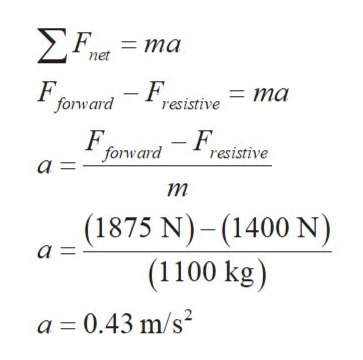# - A boat moves through the water with two forces acting on it. One is a 1,875-N forward push by the water on the propeller, and the other is a 1,400-N resistive force due to the water around the bow.(a) What is the acceleration of the 1,100-kg boat?(b) If it starts from rest, how far will the boat move in 17.5 s?(c) What will its velocity be at the end of that time?

Question
7 views

- A boat moves through the water with two forces acting on it. One is a 1,875-N forward push by the water on the propeller, and the other is a 1,400-N resistive force due to the water around the bow.

(a) What is the acceleration of the 1,100-kg boat?

(b) If it starts from rest, how far will the boat move in 17.5 s?

(c) What will its velocity be at the end of that time?

check_circle

Step 1

Consider two opposite forces are acting on the boat given by  Fforward and Fresistive and their magnitudes are 1875 N and 1400 N respectively.

(a)  Write newton’s second law and plug the required values to find the acceleration of the both, (given mass, m of the boat is 1100 kg).help_outlineImage Transcriptionclose>F ma net F forward -Fresistive ma - F resistive F forward a = т (1875 N)-(1400 N) а - (1100 kg) a 0.43 m/s2 fullscreen
Step 2

(b) Consider boat starts from rest(that is, u=0 m/s), write the motion’s equation and plug t...

### Want to see the full answer?

See Solution

#### Want to see this answer and more?

Solutions are written by subject experts who are available 24/7. Questions are typically answered within 1 hour.*

See Solution
*Response times may vary by subject and question.
Tagged in

### Kinematics